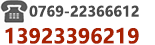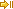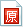﻿ LINQ 主从表查询：同时设置主表及明细表查询条件注意事项|C/S框架网C/S框架网 | 网站导航 | C/S框架文档 - 综合区 | LINQ 主从表查询：同时设置主表及明细表查询条件注意事项
 LINQ 主从表查询：同时设置主表及明细表查询条件注意事项 作者:C/S框架网|www.csframewo  发布日期:2023/03/14 09:44:01LINQ 主从表查询：同时设置主表及明细表查询条件注意事项参考C#代码C# Code:/// /// 库存盘点查询功能/// public List Query(QueryIC input){  var q = _Database.GetQueryable(); //主表   var d = _Database.GetQueryable(); //明细表    //主表查询条件   if (!String.IsNullOrWhiteSpace(input.CreatedBy))  q = q.Where(w => w.CreatedBy == input.CreatedBy);    if (input.DocDateFrom > Globals.MinSqlDate)  q = q.Where(w => w.DocDate >= input.DocDateFrom);    if (input.DocDateTo > Globals.MinSqlDate)  q = q.Where(w => w.DocDate <= input.DocDateTo);    if (!String.IsNullOrWhiteSpace(input.DocNo))  q = q.Where(w => w.ICNO == input.DocNo);    if (!String.IsNullOrWhiteSpace(input.DocNoFrom))  q = q.Where(w => w.ICNO.CompareTo(input.DocNoFrom) >= 0);    if (!String.IsNullOrWhiteSpace(input.DocNoTo))  q = q.Where(w => w.ICNO.CompareTo(input.DocNoTo) <= 0);    //明细表查询条件   if (!String.IsNullOrWhiteSpace(input.StockCode))  d = d.Where(w => w.ProductCode.CompareTo(input.StockCode) == 0);    //获取主表单据号码   var join = (from a in q  join b in d on a.ICNO equals b.ICNO into tmp //LEFT JOIN   from b in tmp.DefaultIfEmpty()  select a);    //返回数据   return join.Distinct().OrderByDescending(o => o.CreationDate).ToList();}//来源:C/S框架网 | www.csframework.com | QQ:23404761注意事项1. 查询条件太多，要定义查询参数模型2. 设置主表查询条件3. 设置明细表查询条件4. 主从表关联，要使用LEFT JOIN, 避免明细表无记录情况下主表出不来5. 要使用DISTINCT语法，因为主从表JOIN，主表记录会根据明细表的数量重复扫一扫加作者微信